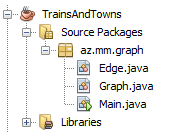Alqoritm

“Trains and Towns” – qraflar üzrə məsələ

Problemin təsviri:

The local commuter railroad services a number of towns in Azerbaijan. Because of monetary concerns, all of the tracks are ‘one-way.’ That is, a route from `A` to `B` does not imply the existence of a route from `B` to `A`.
In fact, even if both of these routes do happen to exist, they are distinct and are not necessarily the same distance!

The purpose of this problem is to help the railroad provide its customers with information about the routes.
In particular, you will compute the

• distance along a certain route,
• the number of different routes between two towns,
• and the shortest route between two towns.

Input: A directed graph where a node represents a town and an edge represents a route between two towns. The weighting of the edge represents the distance between the two towns. A given route will never appear more than once, and for a given route, the starting and ending town will not be the same town.

Output: For test input 1 through 5, if no such route exists, output `'NO SUCH ROUTE'`. Otherwise, follow the route as given; do not make any extra stops! For example, the first problem means to start at city `A`, then travel directly to city `B` (a distance of 5), then directly to city `C` (a distance of 4).

1. The distance of the route `A-B-C`.
2. The distance of the route `A-D`.
3. The distance of the route `A-D-C`.
4. The distance of the route `A-E-B-C-D`.
5. The distance of the route `A-E-D`.
6. The number of trips starting at `C` and ending at `C` with a maximum of `3` stops. In the sample data below, there are two such trips: `C-D-C` (2 stops) and `C-E-B-C` (3 stops).
7. The number of trips starting at `A` and ending at` C` with exactly `4` stops. In the sample data below, there are three such trips: `A` to `C` (via `B``C``D`); `A` to `C` (via `D``C``D`);
and `A` to `C` (via `D``E``B`).
8. The length of the shortest route (in terms of distance to travel) from `A` to `C`.
9. The length of the shortest route (in terms of distance to travel) from `B` to `B`.
10.The number of different routes from `C` to `C` with a distance of less than `30`. In the sample data, the trips are:
`CDC`,` CEBC`,` CEBCDC`,` CDCEBC`,` CDEBC`,` CEBCEBC`,` CEBCEBCEBC`.

Test Input:
For the test input, the towns are named using the first few letters of the alphabet from `A` to `E`. A route between two towns (`A` to `B`) with a distance of `5` is represented as `AB5`.
`Graph: AB5`,` BC4`,` CD8`,` DC8`,` DE6`,` AD5`,` CE2`,` EB3`,``` AE7 ```

Expected Output:
Output #1:    `9`
Output #2:    `5`
Output #3:    `13`
Output #4:    `22`
Output #5:    `NO SUCH ROUTE`
Output #6:    `2`
Output #7:    `3`
Output #8:    `9`
Output #9:    `9`
Output #10:  `7`

Problemin həlli:

Kod strukturu:`Edge.java`

`Graph.java`

`Main.java`